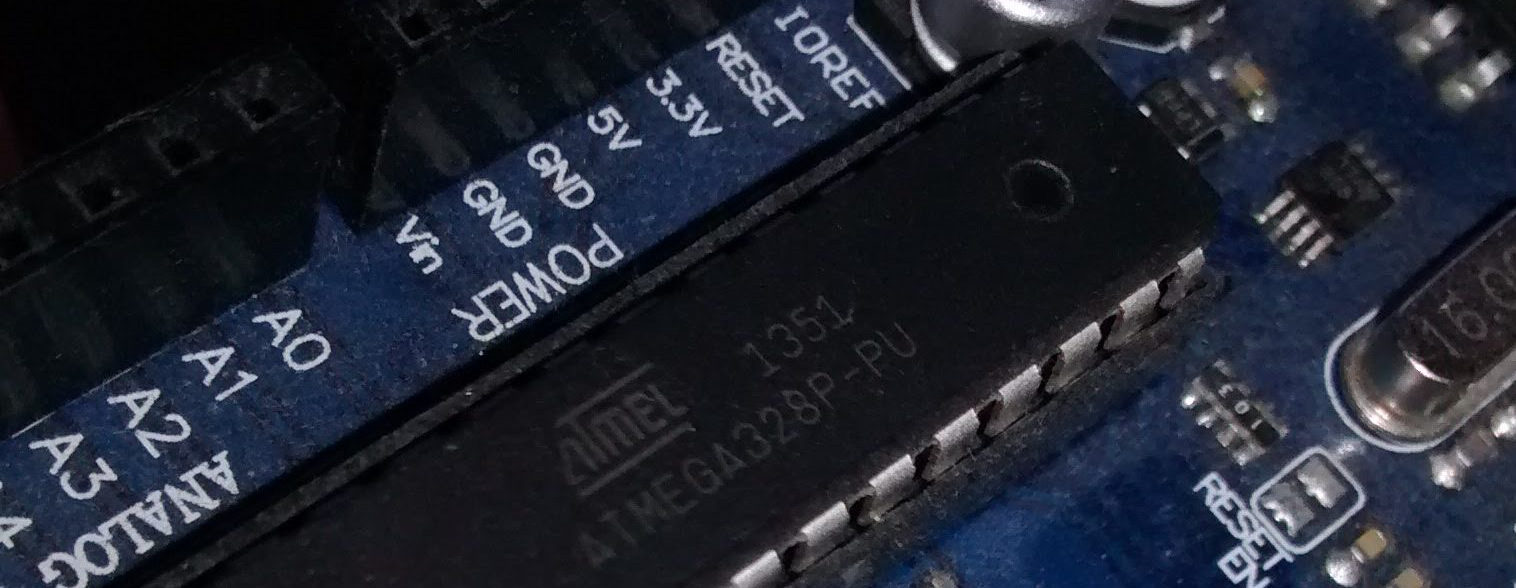# Port Manipulation and Arduino’s digitalWrite Performance

· Timo Denk

The widely used Arduino IDE offers many easy-to-use functions, one of them is  void digitalWrite(uint8_t pin, uint8_t val) . It sets one of the microcontroller’s pins to either high or low and serves well in many cases. However, it has a really poor performance, i.e. execution time. This post analyses both, speed and interior of the digitalWrite function, and proposes alternative, high performance solutions for setting output pins.

### digitalWrite

The source code of Arduino’s digitalWrite function can be found in the Arduino install directory within the path Java/hardware/arduino/avr/cores/arduino in the  wiring_digital.c file.

void digitalWrite(uint8_t pin, uint8_t val)
{
uint8_t timer = digitalPinToTimer(pin);
uint8_t port = digitalPinToPort(pin);
volatile uint8_t *out;

if (port == NOT_A_PIN) return;

// If the pin that support PWM output, we need to turn it off
// before doing a digital write.
if (timer != NOT_ON_TIMER) turnOffPWM(timer);

out = portOutputRegister(port);

uint8_t oldSREG = SREG;
cli();

if (val == LOW) {
*out &= ~bit;
} else {
*out |= bit;
}

SREG = oldSREG;
}


Obviously, there are a lot of checks involved, making the function very robust. Nevertheless, the function’s robustness is not necessarily needed e.g. when the parameter pin is known to be always within $[0,13]$ (Arduino Uno digital pins), or PWM pins are not in use. In such cases several checks can be dropped, leading to a more lightweight version, which works only for the digital pins (PORTB and PORTD). Garretlab has written an article in which the function’s source code is analysed in greater depth.

### digitalWriteFast

void digitalWriteFast(uint8_t pin, uint8_t x) {
if (pin / 8) { // pin &gt;= 8
PORTB ^= (-x ^ PORTB) & (1 &lt;&lt; (pin % 8));
}
else {
PORTD ^= (-x ^ PORTD) & (1 &lt;&lt; (pin % 8));
}
}


digitalWriteFast is a custom function which I wrote. It takes two parameters, just as digitalWrite does:

• uint8_t pin is an integer $\in[0,13]$, standing for the desired digital pin of the Arduino.
• uint8_t x is the second parameter which has only two legal values, namely ${0,1}$. The value $0$ is equivalent to low and $1$ to high. Any other value might alter other pins too, so be aware.

The fast function performs about $2.86\times$ faster and has only been tested on the Arduino Uno. If this function is still too slow, port manipulation could help.

### Port Manipulation

There is a great introduction about the topic on the Arduino website. In a nutshell what it is about, is setting output pins directly, according to the data sheet. This is the fastest possible method of manipulating registers and hence output pin states.

## BenchmarksThe following code was used for the benchmarks. Note that the port manipulation occurs twice: (1) Setting the entire port at once with PORTB = 0; which is usually not desired and (2) setting/clearing just a single bit of a given port.

// setting the entire port
PORTB = 0;
PORTB = 1;

// setting a single bit
PORTB &= ~1;
PORTB |= 1;

// digitalWriteFast
digitalWriteFast(8, 0);
digitalWriteFast(8, 1);

// digitalWrite
digitalWrite(8, LOW);
digitalWrite(8, HIGH);


$t$: Time from low to high (or equivalently high to low)
$f=16\text{MHz}$: Clock speed
$n$: Clock cycles

digitalWrite digitalWriteFast Port manipulation (single bit) (entire port)
$t$ $5\text{µs}$ $1.75\text{µs}$ $0.125\text{µs}$ $62.5\text{ns}$
$n$ $80$ $28$ $2$ $1$
$\times$ $1$ $2.86$ $40$ $80$

## Conclusion

Arduino’s default function digitalWrite does a really good job. It is very robust and easy to use, and should therefore be preferred over any alternative, unless it is too slow.  digitalWriteFast is nearly three times faster but has to be used carefully. Wrong parameter values cause undesired behavior which is incredibly hard to debug. Opposed to the plain bit manipulation, certainly the fasted possible method with just two clock cycles per signal edge, the digitalWriteFast function has the comfortable trait that the pins are chosen based on their number (0 to 13 for the Uno). To sum it up it can be said that the lower-level functions make only sense to use, if performance requirements make it a necessity.